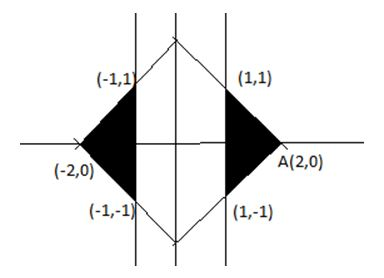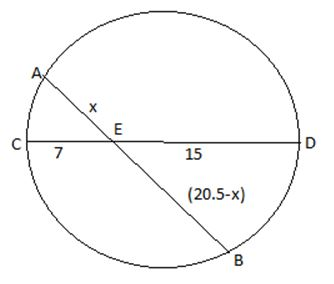### CAT 2019 – Slot 1 – Quantitative Ability – Let S be the set of all points (x, y) in the x-y plane such that

Q. 1: Let S be the set of all points (x, y) in the x-y plane such that | x | + | y | ≤ 2 and | x | ≥ 1. Then, the area, in square units, of the region represented by S equals

The graph of the two functions can be drawn as below:Area of the shaded region = 2*(area of one shaded region) = 2*1/2 *1*2 = 2 unit

##### Q. 2: Ramesh and Gautam are among 22 students who write an examination. Ramesh scores 82.5. The average score of the 21 students other than Gautam is 62. The average score of all the 22 students is one more than the average score of the 21 students other than Ramesh. The score of Gautam is1.   492.   483.   514.   53

Let the score of Gautam =x

Total marks of all the students = 21*62 +x

As per question The average score of all the 22 students is one more than the average score of the 21 students other than Ramesh,

Or (21*62 +x)/22 -1 = (21*62 +x – 82.5)/21

(21*62 + x -22)/22 = (21*62 +x – 82.5)/21

On solving x =51

Q. 3: At their usual efficiency levels, A and B together finish a task in 12 days. If A had worked half as efficiently as she usually does, and B had worked thrice as efficiently as he usually does, the task would have been completed in 9 days. How many days would A take to finish the task if she works alone at her usual efficiency?

1. 18

2. 12

3. 36

4. 24

let usual efficiency of A = 2a and that of B = b

So total work = 12*(2a+b) = 24a+12b————1)

In 2nd case when efficiency of A is halved and efficiency of B get tripled , new efficiency of A = 2a/2 = a

New efficiency of B = b*3 =3b

Total work = 9(a+3b) = 9a+27b ——————-2)

From eq 1) and 2)

24a+12b = 9a + 27b

15a = 15b

Or a=b

So total work = 24a+12b = 24a+12a = 36a

Time taken by A alone to complete the work = 36a/2a = 18 days

Q. 4: If a(base1), a(base2), … are in A.P., then, 1/√a(base1)+√a(base2) + 1/√a(base2)+√a(base3) + … + 1/√a(base n)+√a(base n + 1) is equal to?

1. n-1/√a(base1)+√a(base n)
2. n/√a(base1)+√a(base n + 1)
3. n-1/√a(base1)+√a(base n – 1)
4. n/√a(base1)-√a(base n + 1)

The best method to this problem is to use hit and trial method , for this put n =1 in the question you will get the first term only option a) is equal to that so option a) is correct.

Alternative method )

As given a(base1) , a(base2) ,a(base3) , a(base4) , ………..,a(base n) are in AP.

So a(base2) – a(base1) = a(base3) – a(base2)= a(base4) – a(base3) = …….= a(base n) – a(base n-1) = d (common difference)

Now 1/(√a1+√a2) + 1/(√a2+√a3) + 1/(√a3+√a4) + 1/(√a4+√a5)+⋯.

= 1/(√a1+√a2)×(√a1-√a2)/(√a1-√a2)+1/(√a2+√a3)×(√a2-√a3)/(√a2-√a3)+1/(√a3+√a4)×(√a3-√a4)/(√a3-√a4)+⋯.+⋯.1/(√(a(n-1))+√an)×(√an-√(a(n+1)))/(√an-√(a(n+1)))

= (√a1-√a2)/(a1-a2 )+(√a2-√a3)/(a2-a3 )+(√a1-√a2)/(a1-a2 )+⋯..+(√an-√(a(n+1)))/(an-a(n+1) )

= (√a1-√a2)/(-d )+(√a2-√a3)/(-d )+(√a3-√a4)/(-d )+⋯..+(√an-√(a(n+1)))/(-d )

= (√a1-√an)/(-d )

= (√a1-√(a(n+1)))/(-d )×(√a1+√(a(n+1)))/(√a1+√(a(n+1)))

= 1/(√a1+√an)× (a(n+1) –a1)/d ——1)

Now as we know a(n+1) = a1 + nd

So a(n+1) – a1= nd

Putting this value of a(n+1) – a1in eq 1)

We get required sum = n/(√a1+√(a(n+1)))

Q. 5: In a circle of radius 11 cm, CD is a diameter and AB is a chord of length 20.5 cm. If AB and CD intersect at a point E inside the circle and CE has length 7 cm, then the difference of the lengths of BE and AE, in cm, is

1.   2.5
2.   3.5
3.   0.5
4.   1.5

As per question following figure can be drawn,As we know AE*BE = CE*DE

X(20.5 –x) = 7*15 = 105

20.5x –x^2 = 105

x^2 -20.5x + 105 = 0

x^2 -10.5x – 10x +105 =0

(x -10)*(x-10.5) =0

So x = 10 or 10.5

Thus if AE = 10 then BE = 10.5 and vice versa

Required difference = (10.5 -10) = 0.5

Inspiring Education… Assuring Success!!

Ghatkopar | Borivali | Andheri | Online